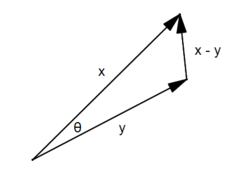## Dot Products and Angles Between Vectors

Suppose we have two $n$-dimensional vectors $x$ and $y$ as shown below:
$$x=\left( \begin{array}{c} x_1 \\ x_2 \\ x_3 \\ \vdots \\x_n \end{array} \right) \textrm{ and } y=\left( \begin{array}{c} y_1 \\ y_2 \\ y_3 \\ \vdots \\ y_n \end{array} \right)$$
We define the dot product of these two vectors, denoted $x \cdot y$ in the following way
$$x \cdot y = x_1 y_1 + x_2 y_2 + x_3 y_3 + \cdots + x_n y_n$$
First, note that we can easily show the dot product is commutative:
$$\begin{array}{rcl} x \cdot y & = & x_1 y_1 + x_2 y_2 \cdots + x_n y_n \\ & = & y_1 x_1 + y_2 x_2 \cdots + y_n x_n \\ & = & y \cdot x \end{array}$$
Almost just as easily, we can show that the dot product distributes over vector sums:
$$\begin{array}{rcl} x \cdot (y + z) & = & x_1 (y_1 + z_1) + x_2 (y_2 + z_2) + \cdots x_n (y_n + z_n)\\ & = & x_1 y_1 + x_1 z_1 + x_2 y_2 + x_2 z_2 + \cdots + x_n y_n + x_n z_n\\ & = & (x_1 y_1 + x_2 y_2 \cdots + x_n y_n) + (x_1 z_1 + x_2 z_2 \cdots + x_n z_n)\\ & = & x \cdot y + x \cdot z \end{array}$$
As one more observation, note that for any scalar $k$, we have
$$\begin{array}{rcl} (kx) \cdot y & = & kx_1 y_1 + kx_2 y_2 + \cdots + kx_n y_n\\ & = & k(x_1 y_1 + x_2 y_2 \cdots + x_n y_n)\\ & = & k(x \cdot y) \end{array}$$
We will use these three properties in our main calculation below.

We also define the norm of a vector $x$, denoted by $||x||$, by
$$||x|| = \sqrt{x_1^2 + x_2^2 + \cdots + x_n^2}$$
This is meant to be geometrically interpreted as the length of the vector, or equivalently, the distance between the points $(0,0,...,0)$ and $(x_1,x_2,...,x_n)$.

Interestingly, we note that this can be written in a much shorter way by invoking the dot product:
$$||x|| = \sqrt{x \cdot x}$$

Now, armed with the ideas of the dot product and the norm of a vector, suppose we are interested in finding the angle between two given vectors, $x$ and $y$.

Recall that the Law of Cosines, a generalization on the Pythagorean Theorem, gives us the relationship between the side lengths of an arbitrary triangle. Specifically, if a triangle has side lengths $a$, $b$, and $c$, then
$$a^2 + b^2 - 2ab\cos \theta = c^2$$
where $\theta$ is the angle between the sides of length $a$ and $b$.

Consider the triangle that can be formed from the vectors $x$, $y$, and $x-y$.Applying the Law of Cosines to this triangle, we have
$$||x||^2 +||y||^2 - 2||x|| \, ||y||\cos \theta = ||x-y||^2$$
But this implies, using our observations about the dot product made above, that
$$\begin{array}{rcl} (x \cdot x) + (y \cdot y) - 2||x|| \, ||y||\cos \theta & = & (x-y) \cdot (x-y)\\ & = & x \cdot (x-y) - y \cdot (x-y)\\ & = & (x \cdot x) - (x \cdot y) - (y \cdot x) + (y \cdot y)\\ & = & (x \cdot x) - (x \cdot y) - (x \cdot y) + (y \cdot y)\\ & = & (x \cdot x) - 2(x \cdot y) + (y \cdot y)\\ \end{array}$$
Subtracting the common $(x \cdot x)$ and $(y \cdot y)$ from both sides, we find
$$- 2||x|| \, ||y||\cos \theta = - 2(x \cdot y)$$
Which, solving for $\cos \theta$ tells us
$$\cos \theta = \frac{x \cdot y}{||x|| \, ||y||}$$